Next: 6.3 Experimental Results in Up: 6 Quantum Diffusion II: Previous: 6.1 Two-Phonon Quantum Diffusion

# 6.2 Muonium Diffusion in KCl

Although nominally outside the terms of reference of this thesis, the following example serves to illustrate the application of the preceding theory, to clear up an historical misunderstanding and to provide continuity with recent studies of muonium diffusion.

Experiments performed by Kiefl et.al.  and MacFarlane et.al.  measured the muonium hop rate in KCl over a wide temperature range, clearly showing the position of the minimum hop rate, characteristic of the crossover from two-phonon limited diffusion to one-phonon activated diffusion, at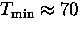K. The measured hop rate below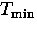was fitted to a power law relation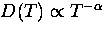, yielding a value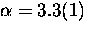.It was noted at the time that the low temperature limiting value of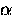predicted by theory was 7 (or 9 in a perfect fcc lattice if the sites are symmetric with respect to the phonon modes); the discrepancy with the measured result remained unexplained.

To answer the question of why the hop rate in KCl did not follow a T-7 dependence, Kagan and Prokof'ev argued in their 1990 paper  that if one used the real phonon spectrum in Eq. (6.8) one could obtain agreement with the measured T-dependence. They wrote also in their review article  that the experimentally found temperature dependence in this region is considerably weaker than that predicted by the limiting law [T-7]. That is, however, associated not with the loss of the dominant role by two-phonon processes but with the real structure of the phonon spectrum of these crystals." Their calculation ofwith the real phonon spectrum in Eq. (6.8) obtained the result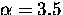.We will show here that their interpretation of the role of structure in the phonon spectrum is not correct. The relative weakness of the temperature dependence (i.e. reduction offrom 7) is a result predicted by this theory, which would be present even if the actual phonon spectrum were entirely Debye-like. The structure present in the phonon density of states of KCl is not responsible for this. Here we will repeat the calculation performed by Kagan and Prokof'ev, and also the case where the phonon spectrum is a featureless, smooth Debye-like function.

The total phonon density of states of KCl, measured by inelastic neutron scattering , is shown in Fig. 6.15. The damping rate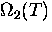can then be calculated using this spectrum for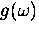in a numerical integration over phonon frequency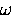.(The Debye temperature of KCl is about 230 K, but the real phonon density of states has its upper cut-off at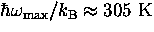.) In this calculation,is normalized so that its integral is unity, which does not affect its T-dependence, only an overall factor. The correct normalization of the phonon density of states can be obtained by calculating the lattice specific heat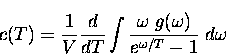(10)

Figure 6.16 shows the temperature dependence of, along with the result obtained if it is assumed that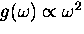.Figure 6.17 shows the temperature dependence of the power law exponent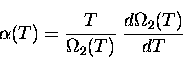for the same cases.

The principal cause of the weak temperature dependence of the muonium hop rate in KCl is that the temperature where the hop rate minimum occurs is already a sufficiently large fraction of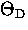that the entire phonon spectrum contributes to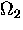. The population of low frequency phonons for which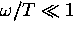increases only linearly with T; the temperature dependence ofdrops off. Since the temperature at which the minimum hop rate occurs is 70 K, a substantial fraction of the Debye temperature, we are far from the low temperature limit where one obtains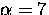.It can be seen from the graph that this is the case whether the real spectrum or a Debye-likeis used. It is an intrinsic property of two-phonon diffusion, not the structure in the spectrum, that causesto be characterized by a lowerat temperatures greater than about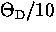or so. The structure in the realhas a small effect on, as can be seen, but the overall shape of the function is hardly affected. A weak temperature dependence of the 2-phonon diffusion rate will occur in any crystal for which(the temperature where the crossover between two-phonon and one-phonon regimes occurs) happens to be more than about, so that two-phonon diffusion is important at relatively high temperatures. Related to this, it must be stressed that the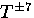dependence is expected only in the low temperature limit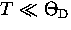.It is a simple exercise to show that in the high temperature limit (several times),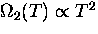.At all intermediate temperatures, the characteristic exponentis a function of temperature, and it is not very meaningful to apply a single value toover a range of temperatures. It is also possible that the coupling to high-frequency modes doesn't follow the simple low frequency limiting behavior. If this is the case, the model will fail completely at higher temperatures.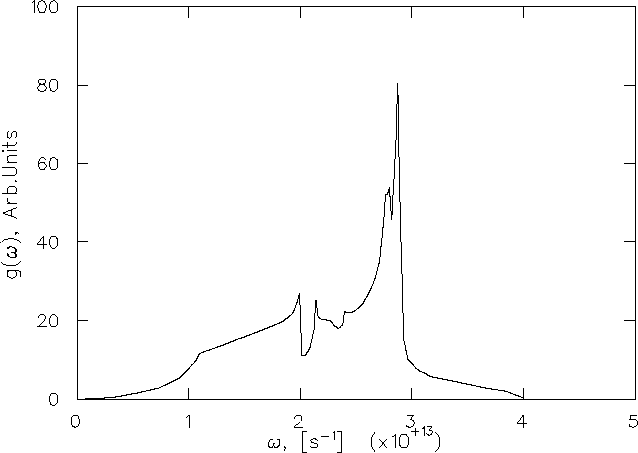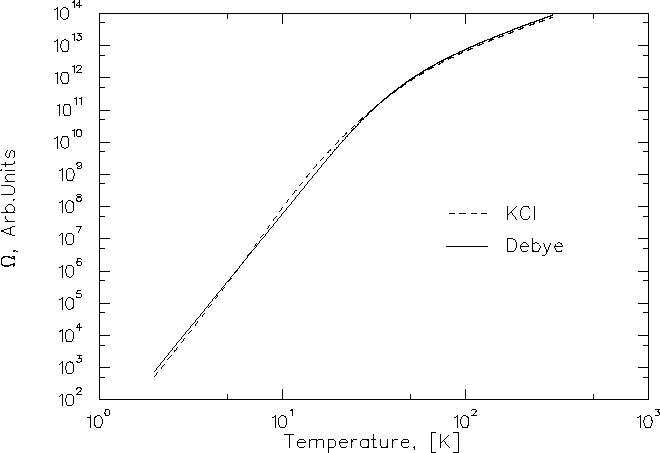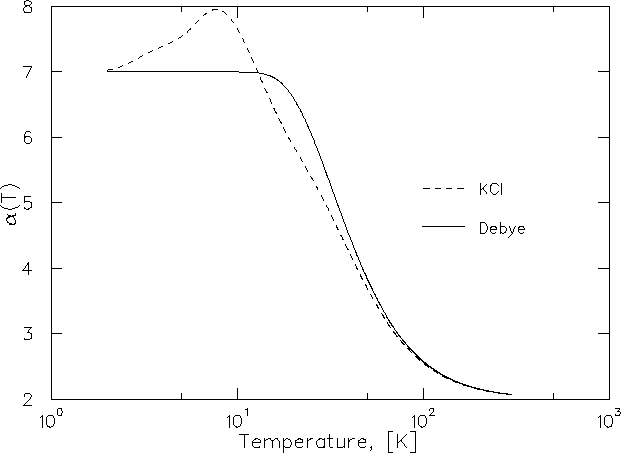Next: 6.3 Experimental Results in Up: 6 Quantum Diffusion II: Previous: 6.1 Two-Phonon Quantum Diffusion